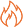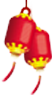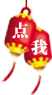# Java编程中的final使用方法

final关键字

1.修饰数据

```class Value {
int v;
public Value(int v) {
this.v = v;
}
}
public class FinalTest {

final int f1 = 1;
final int f2;
public FinalTest() {
f2 = 2;
}
public static void main(String[] args) {
final int value1 = 1;
// value1 = 4;
final double value2;
value2 = 2.0;
final Value value3 = new Value(1);
value3.v = 4;
}
}```

2.修饰方法参数

```public class FinalTest {

/* ... */

public void finalFunc(final int i, final Value value) {
// i = 5; 不能改变i的值
// v = new Value(); 不能改变v的值
value.v = 5; // 可以改变引用对象的值
}
}```

3.修饰方法

4.修饰类Java技术内容HOT资讯 >

• 姓名
• 手机
• 性别
• 意向课程